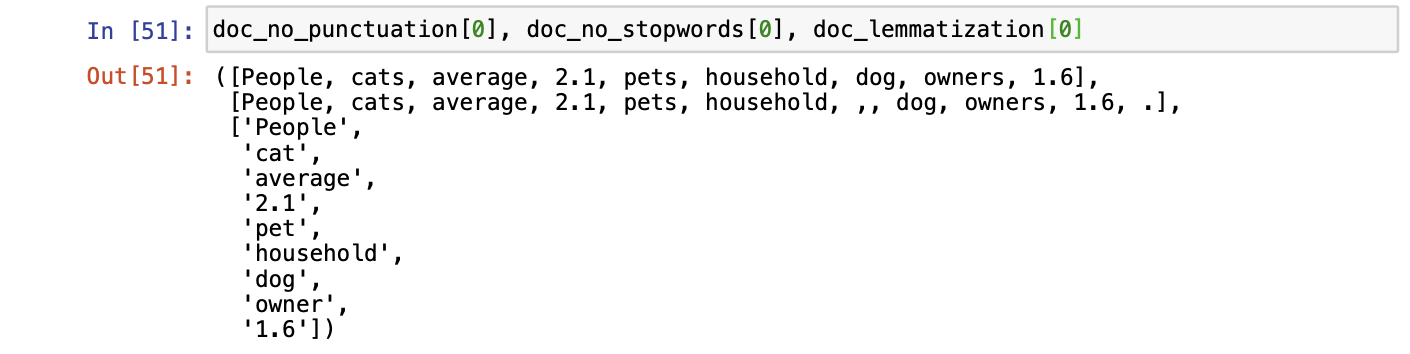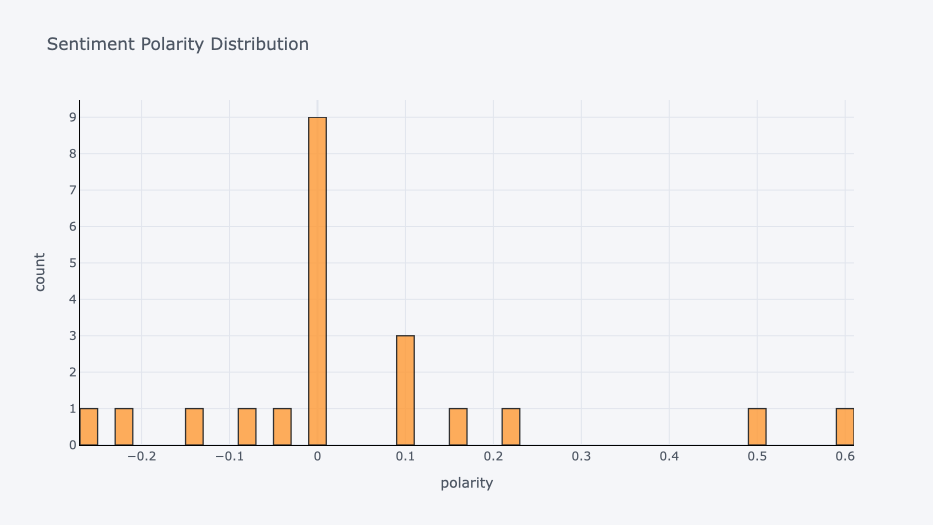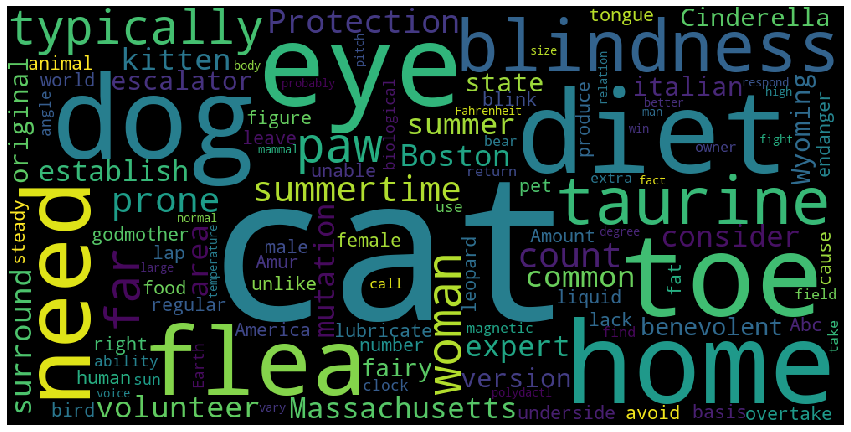# Classifying Random Cat Facts using NLP and Sentiment Analysis

Natural Language Processing using SpaCy and Classifying Random Cat Facts Retrieved Via a Web API Into Positive, Negative or Neutral Using Sentiment Analysis.In this tutorial, we will be retrieving random cat facts using a Web API with the requests package in python.

Next, we will use NLP with spaCy to tokenise, remove stop words and apply lemmatization on our text data.

Finally will use the cleaned data and then perform sentiment analysis using the textblob package to create a score for each fact and to see whether the score is:

• Positive: Score > 0
• Negative: Score < 0
• Neutral: Score = 0

GitHub

The notebook is available on my GitHub. I would suggest cloning the repository for anyone looking to work on the code themselves.

Required Packages

If the package is failing to import, you can resolve the issue using pip e.g.

``````!pip3 install pandas
``````
``````# Import Packages and Libraries
import json
import requests
import pandas as pd
import numpy as np
from textblob import TextBlob
import spacy
from spacy import displacy
from spacy.matcher import Matcher
from urllib.request import urlopen
import plotly.express as px
import matplotlib.pyplot as plt
import itertools
from wordcloud import WordCloud
from collections import Counter

``````

Retrieve Random Cat Facts

The API I am using to retrieve the random cat facts, automatically generates a random fact each time you call the specified URL. Each time it will append the results to responses.

``````def retrieve_cat_facts(url, no_facts):
'''
Retrieve Random Cat Facts Using API call
Append Into Responses
'''
responses = list()
for i in range(0,no_facts):
response = requests.get(f"{url}")
responses.append(data)
return responses, response

responses, response = retrieve_cat_facts(url = 'https://cat-fact.herokuapp.com/facts/random', no_facts = 20)
``````

Output

The function will append all the responses from the function into dictionaries within a list. This is just a snapshot of the first dictionary within the list.You can use the following code below to check that the API call is succesful.

``````if response.status_code == 200:
print('Successful API call')
``````

``````nlp = spacy.load('en')
``````

Create Lists of Lists of ‘Text’ Data

I decided to create a list of lists of all the random cat facts, as we are only concerned with the text data.

``````def create_list_of_lists(number_of_facts):
'''
Create A List Of Lists for the 'text' columns
'''
doc_responses = list()
for x in range (0, number_of_facts):
doc = nlp(responses[x]['text'])
doc_responses.append(doc)
return doc_responses
doc_responses = create_list_of_lists(20)

``````

Length of Sentences

The following code will loop through the words of each sentence, before outputting the number of words in a sentence.

``````def getLengthSentences(number_of_facts, responses):
'''
Get Length of Sentence for each random cat fact
'''
for z in range (0, number_of_facts):
#passing  text into nlp object
sentence = nlp(responses[z]['text'])
#Identify the sentences using attribute
sentences = list(sentence.sents)
for sent in sentences:
print('Sentence: ', sent)
print("The length of the sentences:", len(sent))

getLengthSentences(20, responses)

``````Stop Words

Stop words are the most common words in any NLP model. To analyze text data and build NLP models, these stopwords might not add much value to the meaning of the document. Generally, the most common words used in a text are

• “the”
• “is”
• “in”
• “a”

We will remove the stopwords from our text data before scoring our random cat facts.

``````stopwords = spacy.lang.en.stop_words.STOP_WORDS
# check the length of Stop Words
print("The length of stopwords:", len(stopwords))
for i in list(stopwords)[:20]:
print(i)

``````
``````def remove_stopwords(doc):
'''Remove Stop Words From Text Data'''
final_doc = []
for sentence in doc:
print("Number of tokens in the doc:", len(sentence))
element = []
for word in sentence:
if not word.is_stop:
element.append(word)
final_doc.append(element)
print("Number of tokens after removing stopwords:", len(element))
return final_doc

doc_no_stopwords = remove_stopwords(doc_responses)
``````Remove Punctuation

Generally, you want to remove punctuation from text data, for this project we will remove punctuation. However, there are occasions where punctuation can be used to gain insight into text data.

``````def removePunctuation(doc):
'''
Remove Punctuation From Text Data
'''
final_doc = []
for sentence in doc:
element = []
for word in sentence:
if not word.is_punct:
element.append(word)
final_doc.append(element)
return final_doc
doc_no_punctuation = removePunctuation(final_doc)
``````

Lemmatization

In simpler terms, a method that switches any kind of a word to its base root mode is called Lemmatization.

‘troubled’ -> Lemmatization -> ‘trouble’

‘neglected’ -> Lemmatization -> ‘neglect’

``````def lemmatization(doc):
'''
Apply Lemmatization On Text Data
'''
final_doc = []
for sentence in doc:
element = []
for word in sentence:
element.append(word.lemma_)
final_doc.append(element)
return final_doc

doc_lemmatization = lemmatization(doc_no_punctuation)
``````

Comparison

``````doc_no_punctuation, doc_no_stopwords, doc_lemmatization
``````

From the below image you can see that each of our functions has worked as expected.Part of Speech Tagging

The process of classifying words into their parts of speech and labelling them accordingly is known as part-of-speech tagging, POS-tagging, or simply tagging.

This can be useful within text data to gain a greater overview of what our data represents.The first function converts a ‘list of lists’ -> ‘one list’

``````def changeDataType(doc):
'''Change Data Type into One List for POS'''
final_doc = []
for sentence in doc:
str1 = ' '.join(sentence)
nlp_doc = nlp(str1)
final_doc.append(nlp_doc)
return final_doc

doc_one_list = changeDataType(doc_lemmatization)
``````

The second function returns each word with their POS tag.

``````def pos_tagging(doc):
'''POS Tagging on Text Data'''
doc_pos = []
for sentence in doc:
for word in sentence:
print (word, word.tag_, word.pos_, spacy.explain(word.tag_))
doc_pos.append(word.pos_)
return doc_pos
doc_pos = pos_tagging(doc_one_list)
doc_pos

``````Matcher

SpaCy also offers functionality to match on a pattern, here I have set to match on the words:

• Tom
• Jerry
``````# Import spaCy Matcher
from spacy.matcher import Matcher

# Initialize the matcher with the spaCy vocabulary
matcher = Matcher(nlp.vocab)

matches = []
for i in doc_lemmatization:
print(i)
str2 = ' '.join(i)
doc = nlp(str2)
# Define rule
pattern = [{'TEXT': 'Tom'}, {'TEXT': 'Jerry'}]
matches.append(matcher)
matches_found = matcher(doc)
matches.append(matches_found)
``````
``````# Finding matches and passing the doc to the matches object
matches
``````

You can see from the image below, we have been provided with a unique id, and the respective start element 6 and end element 8 of the matchSentiment Analysis

We now create a score to see how positive or negative each cat fact is, by looping through the sentences and applying sentiment.polarity on each element within the list.

``````score_list = []
for i in doc_lemmatization:
str3 = ' '.join(i)
blob = TextBlob(str3)
for sentence in blob.sentences:
score_list.append(sentence.sentiment.polarity)
print(i,sentence.sentiment.polarity)
``````

You can see the text after NLP next to the score where:

• 1 is the most positive cat fact
• -1 is the most negative cat fact``````df = pd.DataFrame(score_list)
df['score'] = df
del df
df

df['Sentiment'] = np.where(df['score'] == 0,
'Neutral', '')
df['Neutral Flag'] = np.where(df['score'] == 0,
1, 0)
df['Sentiment'] = np.where(df['score'] > 0,
'Positive', df['Sentiment'])
df['Positive Flag'] = np.where(df['score'] > 0,
1, 0)
df['Sentiment'] = np.where(df['score'] < 0,
'Negative', df['Sentiment'])
df['Negative Flag'] = np.where(df['score'] < 0,
1, 0)
df['Sentiment']
df = df[:20]
df
``````

Classifying the data into Positive, Negative and Neutral depending on the score.Data Viz

Being a big fan of Data Viz, what a better way to end with some graphs. I am a big fan of Plotly, but you can adapt the code for your preferred tools.

Pie Chart

``````fig = px.pie(df, names='Sentiment', title='Sentiment Analysis of Random Cat Facts')
fig.update_traces(textposition='inside', textinfo='percent+label')
fig.show()
``````Histogram

``````cf.go_offline()

df['score'].iplot(
kind='hist',
bins=50,
xTitle='polarity',
linecolor='black',
yTitle='count',
title='Sentiment Polarity Distribution')
``````Bar Chart Of Length Of Sentences

``````len_sentence = []
for i in doc_lemmatization:
len_sentence.append(len(i))
=df['len_sentence'] = len_sentence
``````
``````df['len_sentence'].iplot(
kind='hist',
bins=100,
xTitle='word count',
linecolor='black',
yTitle='count',
title='Review Text Word Count Distribution')
``````WordCloud

``````merged = list(itertools.chain(*doc_lemmatization))
#convert list to string and generate
unique_string=(" ").join(merged)
wordcloud = WordCloud(width = 1000, height = 500).generate(unique_string)
plt.figure(figsize=(15,8))
plt.imshow(wordcloud)
plt.axis("off")
plt.show()
plt.close()
``````Bar Chart Of POS Tagging

``````counts = Counter(doc_pos)
common = counts.most_common()
df_pos = pd.DataFrame(common)
df_pos['Count'] = df_pos
df_pos['POS_tagging'] = df_pos

fig = px.bar(df_pos, x='POS_tagging', y='Count', title='Bar Chart of POS Tagging')
fig.show()
``````Conclusion

That’s it for today. I hope you enjoyed this project as much as I have. Whilst there were limitations in the analysis due to the small sample size used, I believe the three things you can take from the project are:

• Introduction to APIs
• Introduction to NLP
• Introduction to Sentiment Analysis

If you have time, you can further explore this project by potentially using the dates and creating a time series based model looking to predict the average sentiment score.##### Shaan Aucharagram
###### Data Science Degree Apprentice

My interests include Quantitative Finance, Machine Learning and Data Viz.